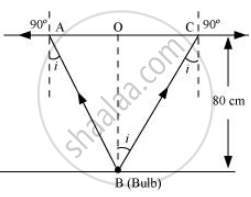# A small bulb is placed at the bottom of a tank containing water to a depth of 80 cm. What is the area of the surface of water through which light from the bulb can emerge out? - Physics

Numerical

A small bulb is placed at the bottom of a tank containing water to a depth of 80 cm. What is the area of the surface of water through which light from the bulb can emerge out? Refractive index of water is 1.33. (Consider the bulb to be a point source.)

#### Solution

Actual depth of the bulb in water, d1 = 80 cm = 0.8 m

Refractive index of water, μ = 1.33

The given situation is shown in the following figure:Where,

i = Angle of incidence

r = Angle of refraction = 90°

Since the bulb is a point source, the emergent light can be considered as a circle of radius, R = "AC"/2 = AO = OB

Using Snell’ law, we can write the relation for the refractive index of water as:

μ = (sin "r")/(sin "i")

1.33 = (sin 90°)/(sin "i")

∴ i = sin^-1 (1/1.33) = 48.75°

Using the given figure, we have the relation:

tan i = "OC"/"OB" = "R"/"d"_1

∴ R = tan 48.75° × 0.8 = 0.91 m

∴ Area of the surface of water = πR2 = π (0.91)2 = 2.61 m2

Hence, the area of the surface of water through which the light from the bulb can emerge is approximately 2.61 m2.

Concept: Snell’s Law
Is there an error in this question or solution?
Chapter 9: Ray Optics and Optical Instruments - Exercise [Page 344]

#### APPEARS IN

NCERT Physics Part 1 and 2 Class 12
Chapter 9 Ray Optics and Optical Instruments
Exercise | Q 9.5 | Page 344
Share